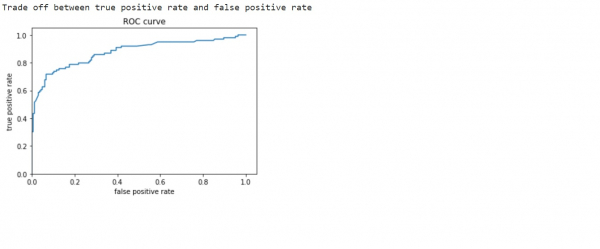# How can Tensorflow and Estimator be used to find the ROC curve on titanic dataset?

Tensorflow and Estimator can be used to find the ROC curve on titanic dataset with the help of ‘roc_curve’ that is present in the ‘sklearn.metrics’ package. The ‘plot’ method plots the data, and the ‘show’ method is used to display this plot on the console.

We will use the Keras Sequential API, which is helpful in building a sequential model that is used to work with a plain stack of layers, where every layer has exactly one input tensor and one output tensor.

A neural network that contains at least one layer is known as a convolutional layer. We can use the Convolutional Neural Network to build learning model.

We are using the Google Colaboratory to run the below code. Google Colab or Colaboratory helps run Python code over the browser and requires zero configuration and free access to GPUs (Graphical Processing Units). Colaboratory has been built on top of Jupyter Notebook.

An Estimator is TensorFlow's high-level representation of a complete model. It is designed for easy scaling and asynchronous training. We will train a logistic regression model using the tf.estimator API. The model is used as a baseline for other algorithms. We use the titanic dataset with the goal of predicting passenger survival, given characteristics such as gender, age, class, etc.

Estimators use feature columns to describe how the model would interpret the raw input features. An Estimator expects a vector of numeric inputs, and feature columns will help describe how the model should convert every feature in the dataset.

## Example

print("Trade off between true positive rate and false positive rate")
from sklearn.metrics import roc_curve
fpr, tpr, _ = roc_curve(y_eval, probs)
plt.plot(fpr, tpr)
plt.title('ROC curve')
plt.xlabel('false positive rate')
plt.ylabel('true positive rate')
plt.xlim(0,)
plt.ylim(0,)
plt.show()

## Output## Explanation

• The receiver operating characteristic (ROC) cuve can be used to visualize the data.

• This helps understand the tradeoff between the true positive rate and false positive rate.